ME - 308 : PROJECT # 2

Design of a Speed Increasing Gear Train

Given Data

Power = 10 KW

Input speed ( n1 ) = 900 rpm

Gear Train Value ( n4 \ n1 ) = 9.375

STEP # 1 : Gear Design

1. Assume Values for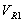and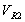such that their product equals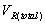2.

Gear Train Value =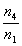=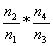==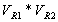= 9.375

3. Solve the two equations for calculating the pitch diameters of the gears 1 and 2 respectively.
4.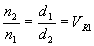-------------------------- ( 1)

Center distance =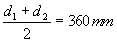--------------------- (2 )

5. Assume a reasonable module ( m):
6. Select a module from table (13-3) in the textbook.

7. Calculate the Number of teeth on the gear # 1 (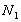):
8.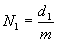This is because, the minimum number for teeth required to avoid interference for gears having a pressure angle of 200 is 18.

If the condition is not satisfied, change m and recalculate the number of teeth on the gear # 1.

9. Calculate the Number of teeth on gear # 2 (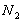) :
10.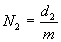Check for the condition that N2>18

11. Calculate the pitch line velocity (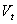) :
12.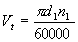m/sec

13. Calculate the Tangential Force (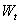) :
14.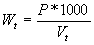N

15. Assume the Face Width of the Gear (F) :
16.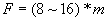mm

17. Calculate the Bending Stress on the gear # 1 (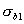) and gear # 2 (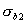) :
18.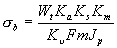Mpa

19. Calculate the Contact Stress on the gear # 1 (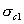) and gear # 2 (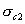) :
20. Note: The contact stress on each of the gears will be equal.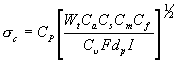MPa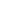21. Select the Materials for the gear # 1 and gear # 2 depending upon the stress values and the Hardness values.
22.

Note: Use the maximum values of the stress and go to the respective figure ( Fig. 14-2 or Fig 14-3) in the text book and select the hardness number and then from the respective table (Tab 14-3 or Tab 14-4) select the material for the gears.

23. Repeat the procedure for the second pair of gears, gear # 3 and gear # 4

BACK